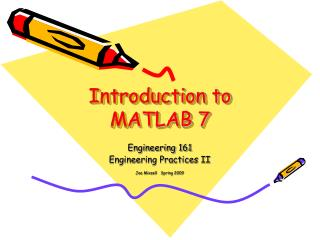# Introduction to MATLAB 7 - PowerPoint PPT PresentationDownload PresentationIntroduction to MATLAB 7

Introduction to MATLAB 7
Download Presentation## Introduction to MATLAB 7

- - - - - - - - - - - - - - - - - - - - - - - - - - - E N D - - - - - - - - - - - - - - - - - - - - - - - - - - -
##### Presentation Transcript

1. Introduction to MATLAB 7 Engineering 161 Engineering Practices II Joe Mixsell Spring 2009

2. MATLAB Properties I • MATLAB is a very powerful mathematical computational tool. • MATLAB comes from MATrix LABoratory • MATLAB provides extensive capabilities for generating graphs • MATLAB is applicable to a broad range of engineering problems

3. MATLAB Properties II • MATLAB supports an interactive and a programming environment • Think of the interactive environment as a “super calculator” with graphics • Think of the programming environment as tool for writing programs to perform complex calculations

4. MATLAB Properties III • Where does MATLAB fit? • It is usually easier to write programs in MATLAB as compared to C or Fortran • C or Fortran programs will usually run faster than MATLAB programs • But, MATLAB is optimized for matrix manipulations and programs formalized in this way will run faster than similar programs in C or Fortran

5. Resources • Text: MATLAB for Engineers • Notes: MATLAB Notes and Assignments • Website: www.engr.uaa.alaska.edu/soe/courses/engr161/ • Website: www.mathworks.com • Student Edition of MATLAB 7 (\$99) • Computer Lab

6. Course of Study • MATLAB Environment Chp. 2 • Built-In MATLAB Functions Chp. 3 • Manipulating MATLAB Matrices Chp. 4 • Plotting Chp. 5 • User Defined Functions Chp. 6 • User Controlled Input and Output Chp. 7 • Logical Functions and Control Structures Chp. 8 • Matrix Algebra Chp. 9 • Symbolic Mathematics Chp. 11 • Polynomials/Curve Fitting Chp. 12

7. MATLAB Assignments • Chp. 2: 2.10, 2.12, 2.13, 2.14 • Chp. 3: 3.3, 3.11, 3.12, 3.16 • Chp. 4: 4.1, 4.7, 4.11 • Chp. 5: 5.3, 5.4, 5.6, 5.17, 5.26 • Chp. 6: 6.4, 6.7, 6.8 • Chp. 7: 7.5, 7.6, 7.9, 7.13, 7.15 • Chp. 8 8.1, 8.2, 8.4, 8.9, 8.16, 8.23, 8.27 • Chp. 9: 9.18, 9.19 • Chp. 11: 11.9, 11.10, 11.17, 11.22, 11.29, 11.30, 11.32

8. Programming Assignment- Project #8 • In this project you will write a user defined function to emulate the mean and standard deviation built in functions. Sorry but you can’t use the built in mean and std functions. You have to develop your own program steps from scratch. This assignment is listed as Project #8.

9. Programming Assignment- Project #9 • This project explores 3-D plotting. You will be determining the electric field strength (E-field) developed by two electric charges lying on a flat surface, then plot the field strength as a function of position. This assignment will give you some familiarity with 3-D plotting and the power of MATLAB to visualize solutions.

10. Assignments • The percent symbol % is used to define a comment, use this following the MATLAB prompt to add your name and assignment number to your work >>% Joe Mixsell >>% Assignment 3.4

11. Assignments • Many assignment require you to print the Edit window, the Command Window and the Figure window(s). Just print them all and staple them together when you turn them in. Remember to clear the command window before your final run to start with a clean slate.

12. More on Assignments • The first few assignments can easily be done with paper and pencil and a calculator but you need to do them using MATLAB. Only outputs from MATLAB will be accepted.

13. An Engineering Problem-Solving Methodology • Step 1: State the problem clearly. • Step 2: Describe the inputs and outputs. • Step 3: Work the problem by hand with a set of simple data. • Step 4: Develop the MATLAB solution • Step 5: Test the solution with real data

14. Getting Started Example 1 d = ½ * g * t^2 where d is the distance a body falls freely in a gravitation field during t seconds. Example 2 x(t) = exp (-alpha * t ) * cos ( omega * t ) i.e, our suspension system we studied previously briefly using Excel

15. Example I • Using MATLAB we want to create a table of values of time and distance for time increments between 0 and 10 seconds.

16. Example II • Using MATLAB we’ll do an analysis of a suspension system and plot position versus time as the system responds to an initial displacement.

17. Continuing with today’s Discussion • MATLAB Help and Demos • Introduce various MATLAB windows • Use the Command Window • Use the Edit Window • View results in the Command Window and the Graphics Window

18. Discussion Next Class- Chapter 2- MATLAB Environment • MATLAB variables • Working with Matrices • Scalar Operations • Precedence of Arithmetic Operators • Array Operations • Saving your Work • Saving Variables • M-files (Writing and saving programs)

19. MATLAB Discussion Sequence • To prepare for the projects we will complete Chapters 2, 3, and 4 then jump to Chapter 6, 7 and 8 for several programming topics (functions, input statements, for loops and if statements ). At this point the first project will be assigned. Then back to Chapter 5 on plotting, continuing from that point.

20. Scheduling our Midterm • Assume we’ll set the midterm date to be in first half of March. It will include Excel, Powerpoint and MATLAB up to that point. • We’ll finalize the date in a couple weeks.

21. And now . . . OFF TO MATLAB… Learn by doing . . .